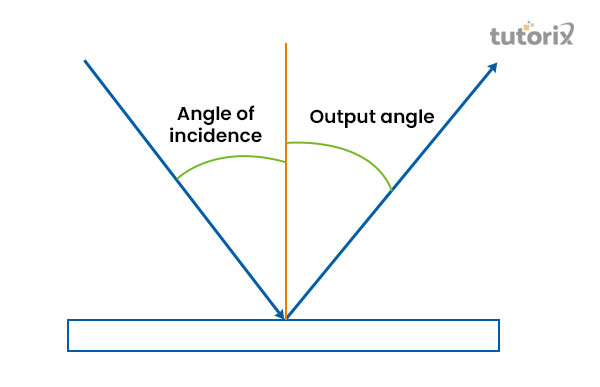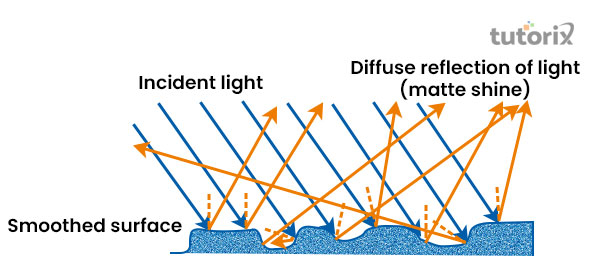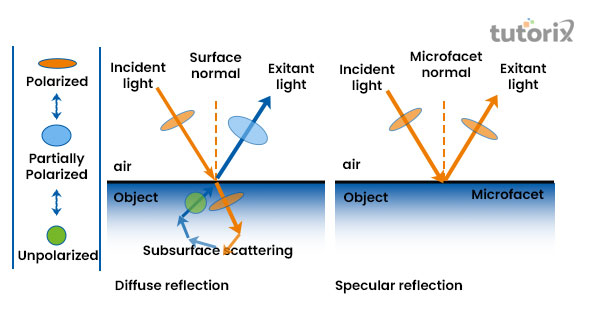# Specular Diffuse Reflection

## Introduction

The act of reflection can be referred to as such kind of phenomenon where the light can be observed to bounce back after being fallen on a particular kind of surface that is considered as the obstacle on the path of the travelling of a ray of light. Based on this concept of physics, the laws of reflection will be discussed in this tutorial. Moreover, the tutorial will include an explanation regarding spectral reflection and diffuse reflection

## What is specular reflection?Figure 1: Specular reflection

The angle of the reflected light is considered similar to the angle of projection of the ray that is projected from a particular source on a surface which is smooth. This phenomenon is generally termed Specular reflection in the terminology of Physics (Minin et al. 2020). Two main factors are determined in order for the occurrence of such kinds of phenomena, such as the surface where the light beams are projected needs to be smooth and the reflected rays will be defused at a similar angle to the projected rays.

## Definition of diffuse reflectionFigure 2: Diffuse reflection

The irregular reflection is generally termed Diffuse reflection in physics which usually takes place on a surface that is not smooth but rather rough enough to scatter the reflected beams.in such cases, it has been observed that the angle of incidence and the reflected beam is not equivalent to each other (Chu et al. 2021). In order to calculate the value of diffuse reflection, the formula can be represented mathematically that is f(R∞)∝(1−R∞)2/R∞.

Laws of reflection

In order to understand the behaviour of light in a specific and predictable manner, on the basis of such a concept, the law of reflection generally states that if a ray of light reflects off a flat mirror, a predictable pattern can be observed in the behaviour of light (Rp-photonics, 2022). The explanation of the laws of reflection is as follows:

It has been seen that the angle of ray that is reflected is equivalent to the angle of the incident. The core condition that must be followed here to observe such a phenomenon is that the surface of reflection is required to be smooth.

The second law of reflection defines that at the contact point with the projected ray, the ray that is reflected is always in the plane, defined by the incident ray and the normal to the surface.

## Difference between Diffuse and Specular reflectionFigure 3: Difference between diffuse and specular reflection

These two main aspects of the reflection have two main differences. The differences behold between these two classifications of reflection are as follows:

• Prior to the explanation of the specular reflection, it has been stated that for the occurrence of this phenomenon, a smooth surface is required and for the diffuse reflection, the surface is considerably rough. Therefore, it can be stated that the main difference lies in the type of surface used for the reflection denoting what kind of reflection will one get (Researchgate, 2022).

• The other difference that is seen is that in the case of specular reflection, the angle of incident ray is equivalent to the angle of reflected beams. On the other hand, the angles that are created by the reflected beams ate not equal to the angle of incident rays.

## Specular and Diffuse reflection: Examples

The most common example of specular reflection is the kind of reflection one can observe in the case of a mirror. The still water surface also acts as a proper surface for witnessing such kind of reflection (Olympus-lifescience, 2022). On the contrary, the Diffuse reflection can be seen at the microscopic level. In addition, if a gust of wind creates a disturbance on the water surface, the water will be responsible for the occurrence of diffusive reflection

## Specular and diffuse reflection: Application

The application of the diffuse reflection is generally observed in the case of transmission measurement as it helps to eliminate the time-consuming process of pressing pallets. It is also used for studying the effect of catalysts and temperature by configuring the accessory with a chamber that is either hot or cool. On the contrary, the specular reflection is applied to get the view of oncoming cars in the side mirrors of cars.

## Conclusion

The present tutorial has focused on defining the spectral reflection and diffuse reflection along with the differences that these two categories of reflection process of light. It has been seen that the core differences these aspects behold are that in the case of Diffuse reflection the reflected beam scatters in different directions as the surface of reflection is rough. On the contrary, while the surface of reflection is plane, the reflected beam of spectral reflection creates a specific angular diversion which is directed towards any one direction.

## FAQs

Q1. Which factors does reflection depend on?

In accordance with the laws of physics, reflection generally depends on the quality of the surface level on what the waves are hitting for creating the reflection. The incident surface is responsible for the change in the angle of the reflection.

Q2. What is the mathematical equation of the specular reflection?

In order to calculate the view direction of the specular reflection, one needs to follow the formula that has a mathematical representation that is Specular≈V.R. In this formula, the letter V is the representative of view direction, and the letter r is considered to be equivalent to 2(N⋅L) N−L.

Q3. What is the difference between regular and diffuse reflection?

The main difference that is shared between regular reflection and diffuse reflection is that the regular reflection is mainly observed on a plane surface whereas; the occurrence of diffuse reflection is observed on a rough surface,

Q4. What results in Diffuse reflection?

A diffuse reflection is responsible for reflecting the rays of light in all directions by scattering them as a tinny amount of imperfection is seen on the surface level of the reflector.

## References

### Journals

Chu, H., Xiong, X., Gao, Y. J., Luo, J., Jing, H., Li, C. Y., ... & Lai, Y. (2021). Diffuse reflection and reciprocity-protected transmission via a random-flip metasurface. Science advances, 7(37), eabj0935. Retrieved from: https://www.science.org

Minin, I. V., Geints, Y. E., Zemlyanov, A. A., & Minin, O. V. (2020). Specular-reflection photonic nanojet: physical basis and optical trapping application. Optics Express, 28(15), 22690-22704. Retrieved from: https://opg.optica.org

## Websites

Olympus-lifescience, (2022). Specular and Diffuse Reflection. Retrieved from: https://www.olympus-lifescience.com [Retrieved on 17th June 2022]